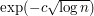## Mathematicians need to be metamathematicians

### Quick description

If you want to prove a theorem, then one way of looking at your task is to regard it as a search, amongst the huge space of potential arguments, for one that will actually work. One can often considerably narrow down this formidable search by thinking hard about properties that a successful argument would have to have. In other words, it is a good idea to focus not just on the mathematical ideas associated with your hoped-for theorem, but also on the properties of different kinds of proofs. This very important principle is best illustrated with some examples.

What can a lower bound say about potential proofs of the upper bound? Brief summary ( There are certain kinds of arguments that always lead to certain kinds of bounds. Therefore, if you are trying to prove a bound, you can often be sure that certain techniques will not be sufficient. This makes the search for techniques that do work more efficient. )

Which techniques lead to which kinds of bounds? Brief summary ( How can a proof ever result in a bound given by a strange-looking function like? This is a good question, and getting to know the answer to it for this and other functions is an important part of the know-how of mathematicians who deal with estimates. Here are links to articles that discuss how various different functions can occur as the end results of proofs.)

When does reformulating a problem count as progress? Brief summary ( Sometimes turning a problem into an equivalent but different-looking problem can result in a statement that is easier to solve. But sometimes it is just a different way of looking at the same problem and results in all the same difficulties. That it should ever help to reformulate a problem may seem rather mysterious. This article investigates the phenomenon. )

If you are getting stuck, then try to prove rigorously that your approach cannot work Brief summary ( Sometimes one gets stuck simply because one has run out of ideas. But sometimes it is for a different reason: one has ideas, but one gets bogged down in technical details and cannot get them to work, or cannot face trying. If that happens to you, it can be very helpful to try to formulate precisely what your approach actually is. Sometimes you will then find that you can make it work, and sometimes you will manage to prove rigorously that it cannot work. (The latter will happen if, for instance, it turns out that you are using a set of assumptions that is not in fact sufficient to imply the conclusion you wish to prove. Then the exercise will tell you that you should try to extract further usable assumptions from your data.) This article contains several examples of approaches to problems that are known not to work. )

Sharp results need sharp lemmas Brief summary ( If you are trying to prove a sharp result, then all the intermediate steps need to be sharp as well. This greatly restricts what they can be, and therefore makes searching for them easier. For this reason it can be a good idea to try to prove a strong result even when all you actually need is a weaker one.)

Two proofs of the same theorem usually have deep similarities Brief summary ( If you are trying to find a new proof of a result, then even if your proposed argument is quite different from others that are known, there are certain features that it is likely to share. In particular, there seems to be a law that one might call conservation of work: at a deep level, any two sensible proofs of the same theorem need the same amount of effort. This can give useful clues about what your new proof is likely to look like. )